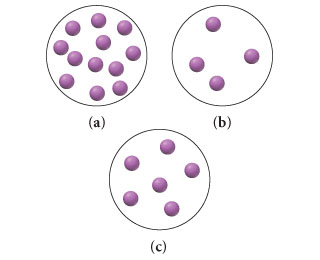# Problem: The circle below represents 1.0 liter of a solution with a solute concentration of 1 M:Explain what you would add (the amount of solute or volume of solvent) to the solution so as to obtain a solution represented by each of the figures (a) - (c)?

###### FREE Expert Solution

We’re being asked to determine what you would add (solute or solvent) to obtain the following solution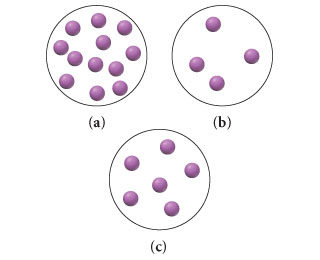Recall that molarity is given by:

Our initial solution contains 8 molecules in a 1.0 L solution. Its concentration is 1 M.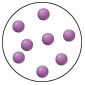This means 1 mole of solute has 8 molecules.

We will do the following steps to solve the problem:

Step 1: Determine the concentration of the final solution

Step 2: Determine the solute or solvent to be added

For a,

Step 1: Determine the concentration of the final solution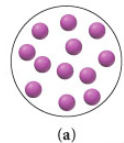91% (42 ratings)###### Problem Details

The circle below represents 1.0 liter of a solution with a solute concentration of 1 M: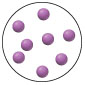Explain what you would add (the amount of solute or volume of solvent) to the solution so as to obtain a solution represented by each of the figures (a) - (c)?